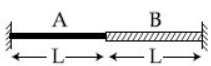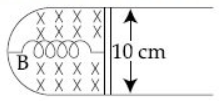## Filters

Sort by :
Clear All
Q

A source  of sound S is moving with a velocity of 50 m/s toward s a stationary observer ,The observer measurs the frequency of the source as 1000Hz .What will be the apparent frequency of the source when it is moving away from the observer after crossing him ? (take velocity of sound in air is 350m/s)

• Option 1)

750 Hz

• Option 2)

857Hz

• Option 3)

1143Hz

• Option 4)

807Hz

Option 1)750 HzOption 2)857HzOption 3)1143HzOption 4)807Hz

The correct figure that shows , schematically , the wave pattern produced by superposition of two waves of frquencies 9 Hz and 11 Hz , is :

• Option 1)• Option 2)• Option 3)• Option 4)Option 1)Option 2)Option 3)Option 4)

A progressive wave travelling along the positive x-direction is represented by . Its snapshot at t=0 is given in the figure.For this wave, the phase  is :

• Option 1)

• Option 2)

• Option 3)

• Option 4)

Travelling Wave Equation -   - wherein   wave length  Time period of oscillation     at  and slope is negative Option 1) Option 2) Option 3) Option 4)

A submarine (A) travelling at  is being chased along the line of its velocity by another submarine (B) travelling at  B sends a sonar signal of  to detect A and receives a reflected sound of frequency . The value of  is close to :

(Speed of sound in water

• Option 1)

• Option 2)

• Option 3)

• Option 4)

frequency of sound when source is moving towards the observer and observer is moving away from source - - wherein Speed of sound Speed of observer speed of source Original frequency apparent frequency     We have given that                                                                                                                                  Frequency received by A,  Frequency...

The displacement of a damped harmonic oscillator is given by

Here t is in seconds. The time taken for its apmlitude of vibration

to drop to half of its initial value is close to:

• Option 1)

• Option 2)

• Option 3)

• Option 4)

Resultant amplitude in damped oscillation - - wherein     amplitude changes from  =>  Option 1) Option 2) Option 3) Option 4)

A stationary source emits sound waves of frequency 500 Hz.

Two observers moving along a line passing through the source

detect sound to be of frequancies 480 Hz and 530 Hz. Their respective speeds

are in m/s,

(Given speed of sound = 300 m/s)

• Option 1)

12,16

• Option 2)

12,18

• Option 3)

16,14

• Option 4)

8,18

Frequency of sound when source is stationary and observer is moving towards source. -   - wherein  speed of sound  Speed of observer  original frequency  apparent frequency     Frequency of sound when source is stationary and observer is moving away from source. - - wherein  speed of sound  Speed of observer  original frequency  apparent...

A damped harmonic oscillator has a frequency of 5 oscillations per second.The amplitude drops to half its value for every 10 oscillations. The time it will take to drop to  of the original amplitude is close to:

• Option 1)

50 s

• Option 2)

100 s

• Option 3)

20 s

• Option 4)

10 s

Resultant amplitude in damped oscillation - - wherein     =5 so  A= So  now at time=t  Option 1) 50 s Option 2) 100 s Option 3) 20 s Option 4) 10 s

A small speaker delivers 2W of audio output. At what distance from the speaker will one detect 120 DB intensity sound?

[Given reference intensity of sound as

• Option 1)

40 cm

• Option 2)

20 cm

• Option 3)

10 cm

• Option 4)

30 cm

Sound level in decibels - - wherein Where is the intensity of the sound and   is a constant reference intensity = 10-12 W/m2     And we know so  Option 1) 40 cm Option 2) 20 cm Option 3) 10 cm Option 4) 30 cm

A tuning fork of frequency 480 Hz is used in an experiment for measuring speed of sound  in air by resonance tube method. Resonance is observed to occur at two successive lengths of the air column,  and . Then,  is equal to:

• Option 1)

332

• Option 2)

384

• Option 3)

338

• Option 4)

379

,     ,            Option 1) 332  Option 2) 384  Option 3) 338  Option 4) 379

Two sources of sounds  and  produce sound waves of the same frequency 660 Hz. A listener is moving from source  towards  with a constant speed u m/s and he hears 10 beats/s. The velocity of sound is 330 m/s. Then, u equals:

• Option 1)

5.5 m/s

• Option 2)

15.0 m/s

• Option 3)

2.5 m/s

• Option 4)

10.0 m/s

apparent frequency received from     apparent frequency received from   beat frequency =           Option 1) 5.5 m/s Option 2) 15.0 m/s Option 3) 2.5 m/s Option 4) 10.0 m/s

A string  long and fixed at its ends is driven by a   vibrator. The string vibrates in its third harmonic mode. The speed of the wave and its fundamental frequency is :

• Option 1)

• Option 2)

• Option 3)

• Option 4)

Option 1) Option 2) Option 3) Option 4)

Two cars A and B are moving away from each other in opposite directions. Both the cars are moving with speed of   with respect to the ground . If an observer in car A detects a frequency   of the sound coming from car B , what is the natural frequency of the sound source in car B ?

• Option 1)

• Option 2)

• Option 3)

• Option 4)

Frequency detected by A = 2000 Hz   Option 1) Option 2) Option 3) Option 4)A wire of length is made by joining two wires A and B of same length but different radii r and 2r and made of the same material. It is vibrating at a frequency such that the joint of the two wires forms a node. If the number of antinodes in wire A is p and that in B is q then the ratio p:q is :

• Option 1)

• Option 2)

• Option 3)

• Option 4)

So Option 1) Option 2) Option 3)   Option 4)

The pressure wave,

corresponds to the sound produced by a vibrating blade on a day when atmospheric temperature is .On some other day when temperature is T. the speed of sound produced by the same blade and at the same frequency is found to be  Approximate value of  is :

• Option 1)

• Option 2)

• Option 3)

• Option 4)

at  at temp            (Where T is in Kelvin)       Option 1)             Option 2) Option 3)      Option 4)

A string is clamped at both the ends and it is vibrating in its  harmonic. The equation of the stationary wave is The length of the string is : (All quantities are in  units.)

• Option 1)

• Option 2)

• Option 3)

• Option 4)

Option 1) Option 2) Option 3) Option 4)

A thin strip 10 cm long is on a U shaped wire of negligible resistance and it is connect to a spring of spring constant (see figure). The assembly is kept in a uniform magnetic field of 0.1 T. If the strip is pulled from its equilibrium position and released, the number of oscillations it performs before its amplitude decreases by a factor of e is N. If the mass of the strip is 50 grams, its resistance and air drag negligible, N will be close to :• Option 1)

10000

• Option 2)

1000

• Option 3)

5000

• Option 4)

50000

for No. of oscillations =Option 1)10000Option 2)1000Option 3)5000Option 4)50000

A simple pendulum oscillating in air has period T, The bob of the pendulum is completely immeresed in a non-viscous liquid.The density of the liquid is of the material of the bob. If the bob is inside liquid all the time,its period of oscillation in this liquid is :

• Option 1)

• Option 2)

• Option 3)

• Option 4)

Option 1)   Option 2)   Option 3)        Option 4)

Equation of sound wave in air is given by. Find the speed of sound in air.

Compare with general equation   Pressure Waves -   - wherein  = Maximum variation in pressure
During simple harmonic motion, Kinetic energy =

A spring of spring constantis stretched initially by  5 cm from the unstretched position.Then the work required to stretch it further by another  5 cm is

Exams
Articles
Questions